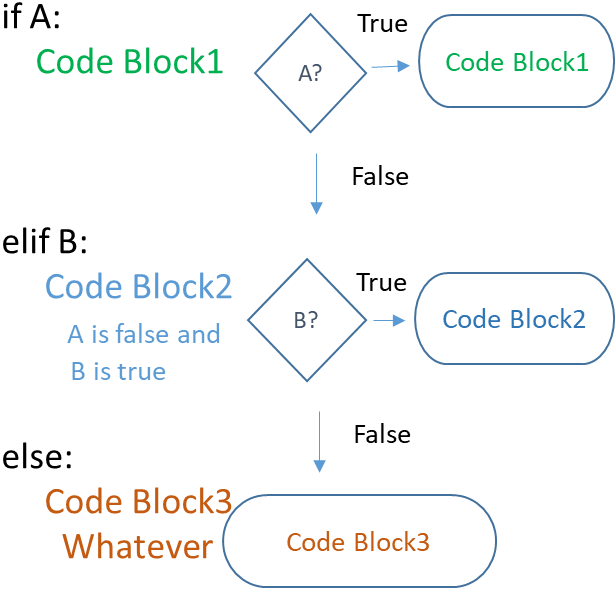# if else elif in Python

Take care of indenting as you will get error. The code to be executed within if condition is to be indented to create a block of code.
``````x=10
y=20
if(x>y):
print("x is greater than y")
else:
print("y is greater than x")``````
Output is here
``y is greater than x``

Python if else and elif to execute code blocks based on condition checking True or False

## Using elif

If the first if condition check fails then we can go for for one more check by using elif before using else.``````x=10
y=20
if(x>y):
print("x is greaeter than y")
elif(y>15):
print("y is greater than 15")

else:
print("y is greater than x but less than 15")``````
Output is here
``y is greater than 15``

## What is the difference between else and elif ?

We don’t check any condition while using else, the code within else block is always executed once the (previous) if condition fails. In case of elif , one more condition is checked.
Read more how to use elif to get the grade from input mark.

## Using Short code

``````x=10
y=20
if(y>x): print("x is greater than y")``````
Output is here
``x is greater than y``
This one is easy.
``````x=10
y=20
print("y is big") if(y>x) else print("x is big")``````
One liner if else
``````mark=45
status='Pass' if mark > 50 else 'Fail'
print(status) # Fail ``````
Write one user defined function to filter odd and even numbers.
``````def my_check(x):
return True if x%2==0 else False``````

## Absolute value of input number

``````i=int(input("input a number "))
if(i<0):i=-i
print("Absolute value is : ",i)``````

## Sort three user input numbers

Using logical operators and, or
``````#n1=int(input('First Number : '))
#n2=int(input('Second Number : '))
#n3=int(input('Third Number : '))
n1=27
n2=18
n3=39
if(n1>=n2 and n1>=n3):
if(n2>=n3):
print(n1,n2,n3)
else:
print(n1,n3,n2)
if(n2>=n1 and n2>=n3):
if(n1>=n3):
print(n2,n1,n3)
else:
print(n2,n3,n1)
if(n3>=n1 and n3>=n2):
if(n1>=n2):
print(n3,n1,n2)
else:
print(n3,n2,n1)``````
``````month='Feb'
if month=='Jan' or month=='Feb' or month=='March':
print("This is last quarter of the Financial year")
else:
print("Financial year is not ending now")``````
Output
``This is last quarter of the Financial year``
Using not
``````grade='A'
print("You are not in grade A ")
else:
print("You are in A grade ")``````
Output
``You are in A grade``

## Check Divisibility

``````i=int(input("Enter any number : "))
if (i%5==0):print("Number is divisible by 5")
else: print("Number is not divisible by 5")``````

Subscribe to our YouTube Channel here

## Subscribe

* indicates required
Subscribe to plus2netplus2net.com